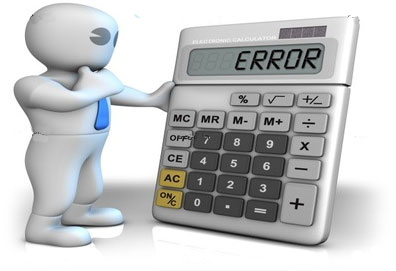# Knowledge Center

## Estimation of Number of Errors. Mills Model. Part I

How many software bugs are in the program? This question worries every programmer. The principle of accumulation of errors gives it a particular relevance, according to it finding an error in a certain module increases the probability that there are other errors this module. It is often impossible to give the exact answer to the question about the number of errors in the program, but to construct an estimate - it is possible. To do this, there are several static models. Let’s consider one of them: Mills Model.In 1972 IBM's super programmer Harlan Mills proposed the following way to estimate the number of errors in the program. Suppose we have a program. Suppose there are N mistakes in it. We call them natural. We insert M artificial errors in addition. We conduct software testing of the program. Suppose that during testing it was found n natural errors and m artificial errors. Suppose that the probability of detection of natural and artificial errors is the same. Then the following is true:

We found the same percentage of natural and artificial errors. Hence the number of errors in the program:

The number of undetected errors is equal to (N - n).

For example, suppose that the program include 20 artificial errors. 12 artificial and 7 natural errors were found during testing. We obtain the following:

The number of undetected errors is equal to (N - n) = 12 - 7 = 5.

TEST MY PROJECT